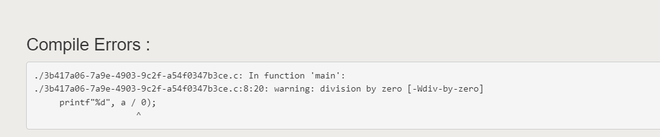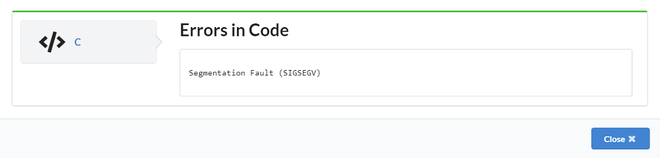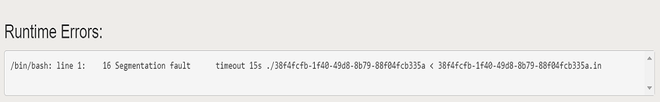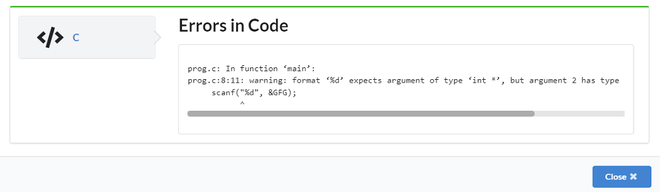# C Program to Show Runtime Exceptions

• Last Updated : 03 Aug, 2022

Runtime exceptions occur while the program is running. Here, the compilation process will be successful.  These errors occur due to segmentation faults and when a number is divided by a division operator or modulo division operator.

## Types of Runtime Errors:

1. An invalid memory access
2. Dividing by zero.
3. Segmentation faults
4. Large memory allocation/Large Static Memory Allocation
5. Making common errors.

### 1. During runtime, an invalid memory access

An invalid memory access error occurs during runtime when the array’s index is assigned a negative value.

Note: The output might change every time we run this program because it gives a junk value in return for the invalid location.

## C

 `// C program to demonstrate``// an invalid memory access error``#include ``int` `a;``int` `main()``{``    ``int` `s = a[-11];``    ``printf``(``"%d"``, s);``    ``return` `0;``}`

Output

`32746`

### 2. Dividing by zero

When we are trying to divide any number by zero then we get this type of error called floating-point errors.

## C

 `// C program to demonstrate``// an error occurred by dividing 0``#include ` `int` `main()``{``    ``int` `a = 5;``    ``printf``(``"%d"``, a / 0);``    ``return` `0;``}`

Output:Error of dividing by zero

### 3. Segmentation Faults

Let us consider an array of length 5 i.e. array, but during runtime, if we try to access 10 elements i.e array then we get segmentation fault errors called runtime errors. Giving only an array length of 5

## C

 `// C program to demonstrate``// an error of segmentation faults``#include ` `int` `main()``{``    ``int` `GFG;``    ``GFG = 10;``    ``return` `0;``}`

But in output trying to access more than 5 i.e if we try to access array during runtime then we get an error

Output:Segmentation Fault Error

### 4. Large memory allocation/Large Static Memory Allocation :

In general, all online judges allow up to 10^8. However, to be on the safe side, use up to 10^7 unless it is required. In the below example we have mentioned more than 10^8 so, it will cause an error due to large memory allocation.

## C

 `// C program to demonstrate``// an error of large memory allocation``#include ` `int` `main()``{``    ``int` `GFG;``    ``printf``(``"%d"``, GFG);``    ``return` `0;``}`

Output:Large Memory Allocation Error

### 5. Making common errors:

The below code gives runtime error because we have declared a variable as long int but in scanf we have used %d instead of %ld. So it will give an error.

## C

 `// C program to demonstrate``// a common error``#include ` `int` `main()``{``    ``long` `int` `GFG;``    ``scanf``(``"%d"``, &GFG);``    ``return` `0;``}`

Output:Common Error

My Personal Notes arrow_drop_up